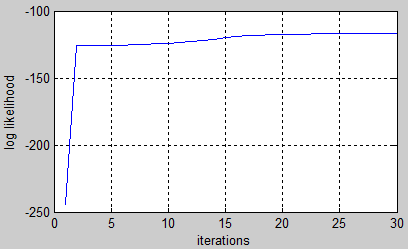2 views

I am having a tough time figuring out how to use Kevin Murphy's HMM toolbox Toolbox. It would be a great help if anyone who has experience with it could clarify some conceptual questions. I have somehow understood the theory behind HMM but it's confusing how to actually implement it and mention all the parameter setting.

There are 2 classes so we need 2 HMMs.

Let say the training vectors are :class1 O1={ 4 3 5 1 2} and class O_2={ 1 4 3 2 4}.

Now, the system has to classify an unknown sequence O3={1 3 2 4 4} as either class1 or class2.

1. What is going to go to obsmat0 and obsmat1?

2. How to specify/syntax for the transition probability transmat0 and transmat1?

3. what is the variable data going to be in this case?

4. Would a number of states Q=5 since there are five unique numbers/symbols used?

5. A number of outputs symbols=5?

6. How do I mention the transition probabilities transmat0 and transmat1?

by (33.1k points)

In this case, the states of the model are the three possible types of weather: sunny, rainy and foggy. We assume the weather can be only one of these values. Thus the set of HMM states are:

S = {sunny, rainy, foggy}

Here, we can't observe the weather directly. Instead, the only evidence we have is whether the person every day is carrying an umbrella or not. In HMM terminology, these are the discrete observations:

x = {umbrella, no umbrella}

The HMM model is determined by three things:

• The prior probabilities: vector of probabilities of being in the first state of a sequence.

• The transition prob: matrix describing the probabilities of going from one state of the weather to another.

• The emission prob: matrix describing the probabilities of observing an output (umbrella or not) given a state (weather).

We may be given with these probabilities, or we have to learn them from a training set. Then, perform reasoning like computing likelihood of an observation sequence with respect to an HMM model

Some steps to follow:

1) known model parameters

Check out this sample code that shows how to fill existing probabilities to build the model:

Q = 3;    # number of states (sun,rain,fog)

O = 2;    # number of discrete observations (umbrella, no umbrella)

#  prior probabilities

prior = [1 0 0];

# state transition matrix (1: sun, 2: rain, 3:fog)

A = [0.8 0.05 0.15; 0.2 0.6 0.2; 0.2 0.3 0.5];

# observation emission matrix (1: umbrella, 2: no umbrella)

B = [0.1 0.9; 0.8 0.2; 0.3 0.7];

Then we can sample a bunch of sequences from this model:

num = 20;           # 20 sequences

T = 10;             # each of length 10 (days)

[seqs,states] = dhmm_sample(prior, A, B, num, T);

For example

>> seqs(5,:)        # observation sequence

ans =

2     2 1     2 1 1     1 2 2 2

>> states(5,:)      # hidden states sequence

ans =

1     1 1     3 2 2     2 1 1 1

Then, evaluate the log-likelihood of the sequence:

dhmm_logprob(seqs(5,:), prior, A, B)

dhmm_logprob_path(prior, A, B, states(5,:))

Compute the Viterbi path (most probable state sequence):

vPath = viterbi_path(prior, A, multinomial_prob(seqs(5,:),B))2) unknown model parameters

Training is performed using the EM algorithm and is best done with a set of observation sequences.

We can use the generated data above to train a new model and compare it to the original:

prior_hat = normalise(rand(Q,1));

A_hat = mk_stochastic(rand(Q,Q));

B_hat = mk_stochastic(rand(Q,O));

# learn from data by performing many iterations of EM

[LL,prior_hat,A_hat,B_hat] = dhmm_em(seqs, prior_hat,A_hat,B_hat, 'max_iter',50);

# plot learning curve

plot(LL), xlabel('iterations'), ylabel('log likelihood'), grid onIn this example, the trained model looks close to the original one:

>> p = [2 3 1];              # states permutation

>> prior, prior_hat(p)

prior =

1     0 0

ans =

0.97401

7.5499e-005

0.02591

>> A, A_hat(p,p)

A =

0.8         0.05 0.15

0.2          0.6 0.2

0.2          0.3 0.5

ans =

0.75967      0.05898 0.18135

0.037482      0.77118 0.19134

0.22003      0.53381 0.24616

>> B, B_hat(p,[1 2])

B =

0.1          0.9

0.8          0.2

0.3          0.7

ans =

0.11237      0.88763

0.72839      0.27161

0.25889      0.74111

To know more about Hidden Markov Model, study Artificial Intelligence Course.